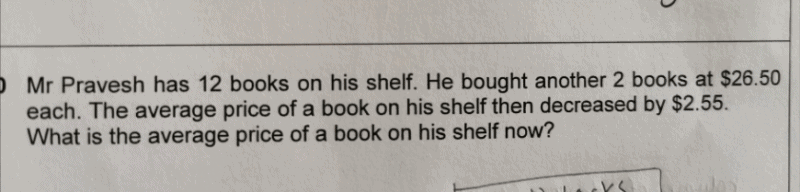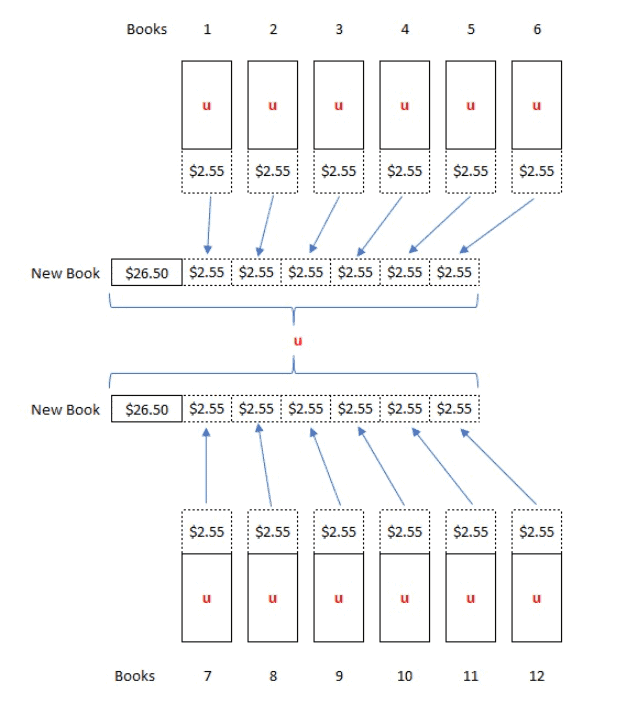# QuestionHi,

Can someone help to explain this qns?

Thanks

Average price of 14 books -> u

Total price of 14 books -> 14u

Average price of 12 books -> u + \$2.55

Total price of 12 books -> 12(u + 2.55) = 12u + 30.6

14u = (12u + 30.6) + (26.50 × 2)

2u = 83.6

u = 41.8

Average of books after adding 2 books at \$26.50 each -> \$41.80

Thank you 😊

0 Replies 0 LikesWhile the problem can be solved by formulating equations as I had done in the first solution, I think it would be good for the child to understand the concept of averages.

When the average decreased by \$2.55 after adding 2 new books, the 12 original books “lost” \$2.55 in value.

These amounts were “added” to the prices of the 2 new books, so that their prices appeared to be same as the other 12.

So, the new average is simply \$26.50 + (6 × \$2.55) = \$41.80

0 Replies 0 Likes